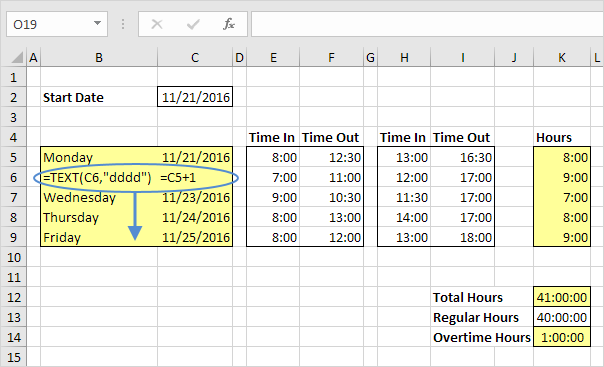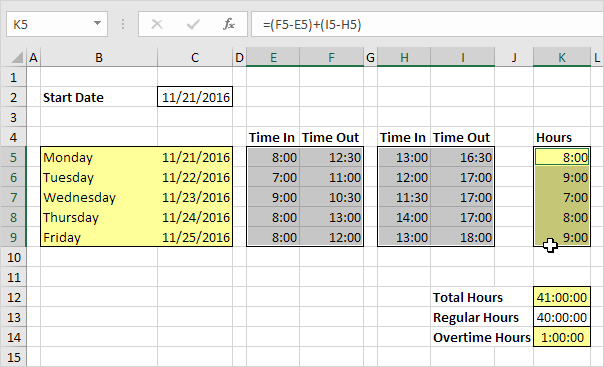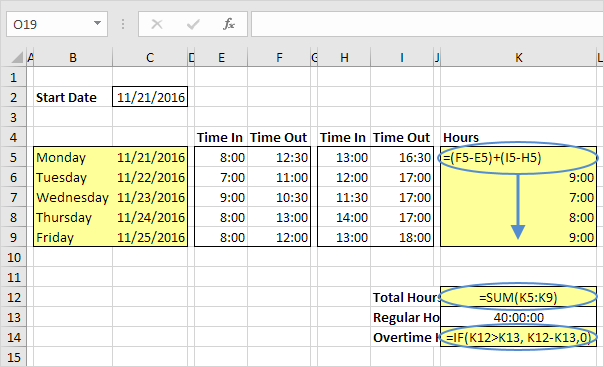## Excel Office

Excel How Tos, Tutorials, Tips & Tricks, Shortcuts

# How to create simple Time Sheet in Excel

This example teaches you how to create a simple timesheet calculator in Excel. Cells that contain formulas are colored light yellow.

1. To automatically calculate the next 4 days and dates when you enter a start date, use the formulas below.Worked Example:   How to calculate percent of goal in Excel

2. Select the cells containing the times.

Worked Example:   How to calculate Quarter of Date in Excel3. Right click, click Format Cells, and select the right Time format. Use the circled format for cell K12, K13, K14.

Worked Example:   Wrap Text Automatically vs Manual Line Break in Excel4. To automatically calculate the hours worked each day, the total hours and the overtime hours, use the formulas below.